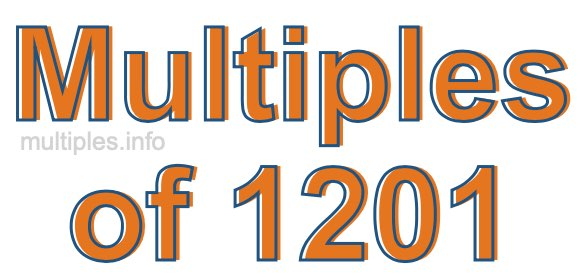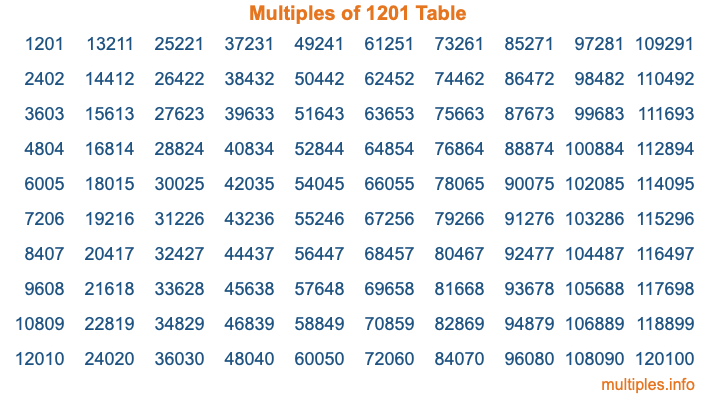Multiples of 1201Welcome to the Multiples of 1201 page. Here we will first teach you everything you will ever need to know about the multiples of 1201, and then give you a study guide summary of everything we taught you to make sure you remember it all. Use this page to look up facts and learn information about the multiples of 1201. This page will make you a multiples of one thousand two hundred one expert!

Definition of Multiples of 1201
Multiples of 1201 are all the numbers that when divided by 1201 equal an integer. Each of the multiples of 1201 are called a multiple. A multiple of 1201 is created by multiplying 1201 by an integer.

Therefore, to create a list of multiples of 1201, you start with 1 multiplied by 1201, then 2 multiplied by 1201, then 3 multiplied by 1201, and so on for as long as you want. Thus, the list of the first five multiples of 1201 is 1201, 2402, 3603, 4804, and 6005. To see a larger list of multiples of 1201, see the printable image of Multiples of 1201 further down on this page. We also have a category where you can choose any nth multiple of 1201.

Multiples of 1201 Checker
The Multiples of 1201 Checker below checks to see if any number of your choice is a multiple of 1201. In other words, it checks to see if there is any number (integer) that when multiplied by 1201 will equal your number. To do that, we divide your number by 1201. If the the quotient is an integer, then your number is a multiple of 1201.

Is  a multiple of 1201?

Least Common Multiple of 1201 and ...
A Least Common Multiple (LCM) is the lowest multiple that two or more numbers have in common. This is also called the smallest common multiple or lowest common multiple and is useful to know when you are adding our subtracting fractions. Enter one or more numbers below (1201 is already entered) to find the LCM.

Check out our LCM Calculator if you need more details about the Least Common Multiple or if you need the LCM for different numbers for adding and subtraction fractions.

nth Multiple of 1201
As we stated above, 1201 is the first multiple of 1201, 2402 is the second multiple of 1201, 3603 is the third multiple of 1201, and so on. Enter a number below to find the nth multiple of 1201.

th multiple of 1201

Multiples of 1201 vs Factors of 1201
1201 is a multiple of 1201 and a factor of 1201, but that is where the similarities end. All postive multiples of 1201 are 1201 or greater than 1201. All positive factors of 1201 are 1201 or less than 1201.

Below is the beginning list of multiples of 1201 and the factors of 1201 so you can compare:

Multiples of 1201: 1201, 2402, 3603, 4804, 6005, etc.

Factors of 1201: 1, 1201

As you can see, the multiples of 1201 are all the numbers that you can divide by 1201 to get a whole number. The factors of 1201, on the other hand, are all the whole numbers that you can multiply by another whole number to get 1201.

It's also interesting to note that if a number (x) is a factor of 1201, then 1201 will also be a multiple of that number (x).

Multiples of 1201 vs Divisors of 1201
The divisors of 1201 are all the integers that 1201 can be divided by evenly. Below is a list of the divisors of 1201.

Divisors of 1201: 1, 1201

The interesting thing to note here is that if you take any multiple of 1201 and divide it by a divisor of 1201, you will see that the quotient is an integer.

Multiples of 1201 Table
Below is an image of the first 100 multiples of 1201 in a table. The table is in chronological order, column by column. The first column has the first ten multiples of 1201, the second column has the next ten multiples of 1201, and so on.The Multiples of 1201 Table is also referred to as the 1201 Times Table or Times Table of 1201. You are welcome to print out our table for your studies.

Negative Multiples of 1201
Although not often discussed or needed in math, it is worth mentioning that you can make a list of negative multiples of 1201 by multiplying 1201 by -1, then by -2, then by -3, and so on, to get the following list of negative multiples of 1201:

-1201, -2402, -3603, -4804, -6005, etc.

Multiples of 1201 Summary
Below is a summary of important Multiples of 1201 facts that we have discussed on this page. To retain the knowledge on this page, we recommend that you read through the summary and explain to yourself or a study partner why they hold true.

There are an infinite number of multiples of 1201.

A multiple of 1201 divided by 1201 will equal a whole number.

1201 divided by a factor of 1201 equals a divisor of 1201.

The nth multiple of 1201 is n times 1201.

The largest factor of 1201 is equal to the first positive multiple of 1201.

1201 is a multiple of every factor of 1201.

1201 is a multiple of 1201.

A multiple of 1201 divided by a divisor of 1201 equals an integer.

1201 divided by a divisor of 1201 equals a factor of 1201.

Any integer times 1201 will equal a multiple of 1201.

Multiples of a Number
Here you can get the multiples of another number, all with the same attention to detail as we did for multiples of 1201 on this page.

Multiples of
Multiples of 1202
Did you find our page about multiples of one thousand two hundred one educational? Do you want more knowledge? Check out the multiples of the next number on our list!